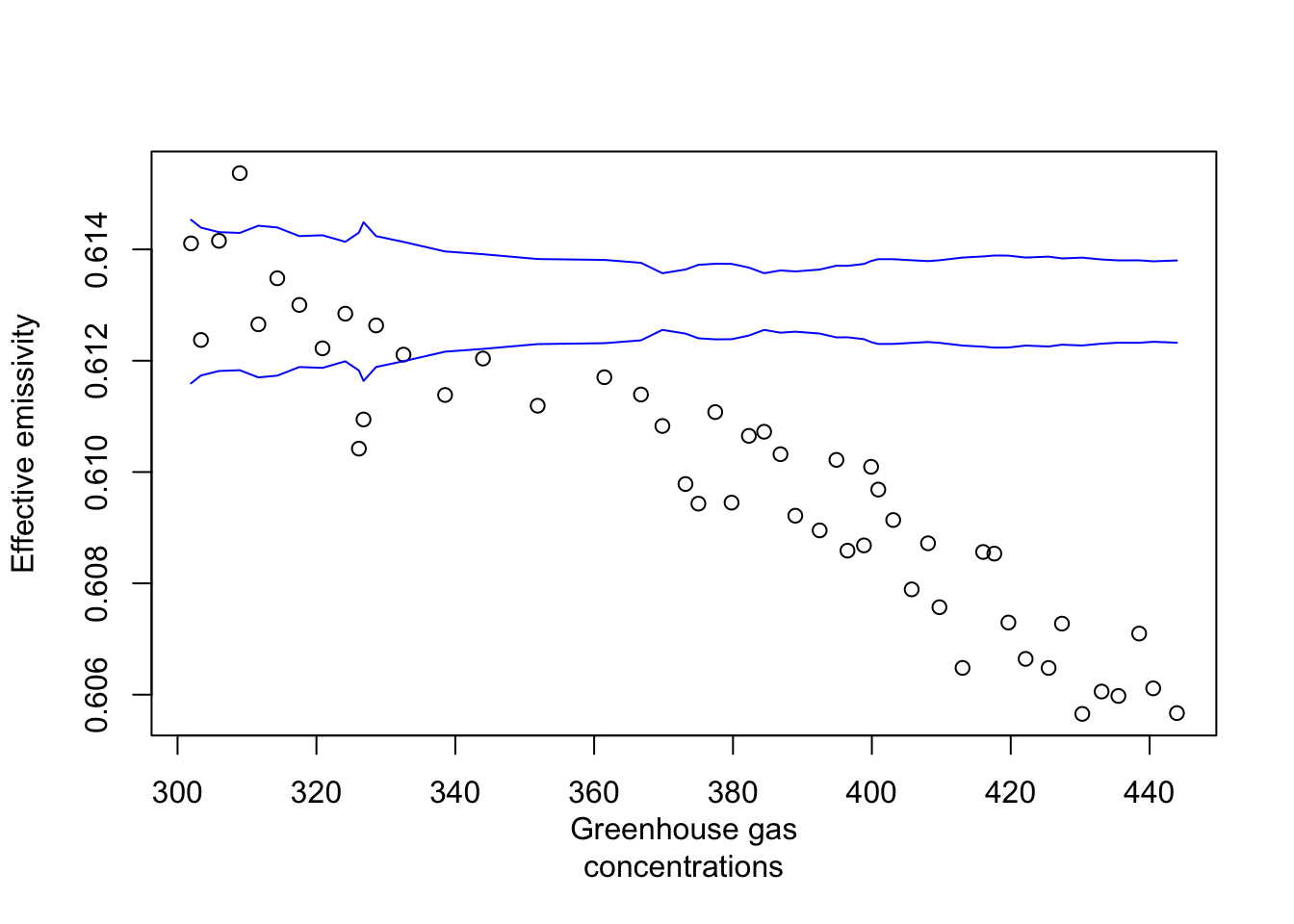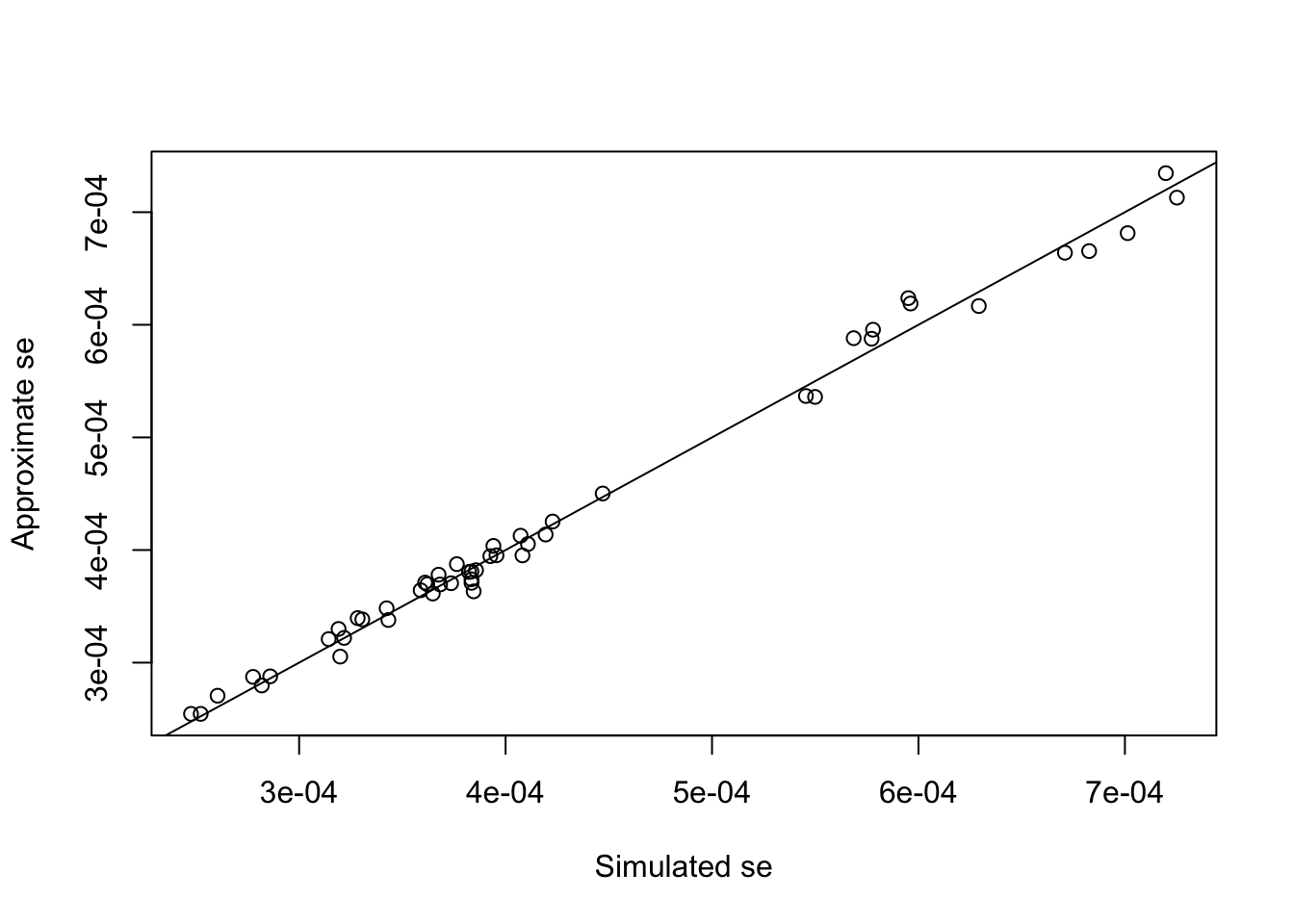Kirkhoff’s law (a zero-dimensional climate model) says the energy coming in, $S \pi r^2 (1-a)$ where $$S$$ is the solar constant (radiation reaching earth in W/m$$^2$$) and $$a$$ is the albedo, equals the energy going out, $4 \pi r^2 \epsilon \sigma T^4$ where $$\sigma$$ is Stefan’s constant 5.67e-08 W/(K$$^4$$m$$^2$$) and $$\epsilon$$ is the emissivity, which we will estimate from observed temperature.

# Setup values

sigma=5.67036713e-08
a=0.3 #current albedo 

berkeley=read.table("~/Dropbox/Klima/Berkeley_Land_and_Ocean_summary.txt")
ber=list(mean=NULL,sd=NULL,years=NULL)
ber$mean=berkeley[,2] ber$years=berkeley[,1]
ber$sd=berkeley[,3] ber$mean=ber$mean+14.764+273.15 #Change from deg C anomalies to K ghg=read.csv("~/Dropbox/Klima/Figure 1 Observed trends in the Kyoto Gases concentrations 2013.csv") names(ghg)=c("Year","CO2","AllGHG") tsi=read.table("~/Dropbox/Klima/TIM_TSI_Reconstruction.txt",skip=7) names(tsi)=c("Year","TSI") tsi$Year=floor(tsi$Year) # Determine indexes for common years elements=which(is.element(ber$years,intersect(ghg[,1],ber$years)))[-(1:9)] #Removing pre-1800 observations ghgels=which(is.element(ghg[,1],intersect(ghg[,1],ber$years)))[-(1:9)]

years_to_use=ghg[ghgels,1]
tsiels=which(is.element(tsi[,1],intersect(tsi[,1],years_to_use))) 

emissivity=tsi[tsiels,2]*(1-a )/4/sigma/ber$mean[elements]^4 preind=mean(emissivity[1:10])#pre 1850 ense=4*emissivity*ber$sd[elements]/ber$mean[elements]#se computed from Taylor expansion  # Plot greenhouse gases vs. estimated emissivity plot(ghg$AllGHG[ghgels],emissivity,xlab="Greenhouse gas
concentrations",ylab="Effective emissivity")
lines(ghg$AllGHG[ghgels],preind-2*ense,col="blue") lines(ghg$AllGHG[ghgels],preind+2*ense,col="blue")The lines are $$\pm2$$ standard errors around the preindustrial emissivity (corresponding to an average temperature of 14.3607deg C). We conclude that the observed temperature is not consistent with the hypothesis of a preindustrial (natural) greenhouse gas forcing.

We can check the standard error by simulating the temperature series:

nrep=1000
emp=matrix(NA,nrow=nrep,ncol=length(elements))
for (i in 1:nrep) {temp=rnorm(length(ber$mean[elements]),ber$mean[elements],ber\$sd[elements])
emp[i,]=tsi[tsiels,2]*(1-a )/4/sigma/temp^4 }
plot(apply(emp,2,sd),ense,xlab="Simulated se",ylab="Approximate se")
abline(0,1)We see that the standard error looks fine.

There is little data suggesting more than a very minor change in albedo. These are mainly based on satellite measurements, and thus do not reach very far back in time.